# Tubing Length Change due to Ballooning

Ballooning is a change in average pressure which causes a radial contraction or swelling. If the tubing is free to move, the length of tubing will be either longer or shorter. If tubing cannot be moved, stress will be created in the tubing body.

Figure 1 illustrates ballooning of tubing. This will happen when internal pressure is higher than external pressure. Tubing will shorten if the tubing is free to move. If the tubing cannot be moved, ballooning will create a tension force on the packer.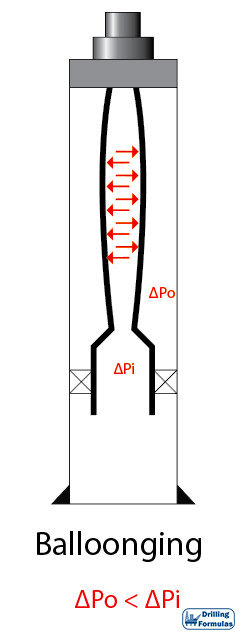Figure 1 – Ballooning

Figure 2 illustrates reverse ballooning of tubing. This will happen when external pressure is higher than internal pressure. Tubing will lengthen if the tubing is free to move. If the tubing cannot be moved, ballooning will create a compressive force on the packer.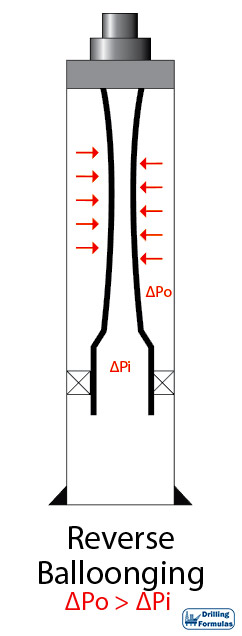Figure 2 – Reverse Ballooning

Ballooning effect is directly related to an area that pressure acts upon. The outside area of tubular is bigger than the inside area of tubular. Therefore, a change in annulus pressure has a greater effect than a corresponding change in tubing. When considering about ballooning, the average pressure along the tubing string is used instead of localized pressure.

The cause of ballooning and reverse ballooning is due to a change in pressure from the initial condition, not differential pressure between tubing and annulus at a particular time. Hence, the tubing length change calculation due to ballooning is based on the average pressure change in tubing and annulus.

Length Change due to Ballooning Formula (If tubing is free to move)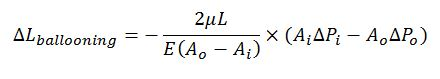Force due to ballooning if the tubing cannot be freely moved.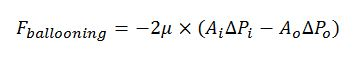Where;

Ai = Tubing ID area (in2)

Ao = Tubing OD area (in2)

L = Length of tubing (in)

ΔPo = Change in average annulus pressure

ΔPi = Change in average tubing pressure

ΔFballooning =Change in force (lb)

ΔLballooning = Change in length due to ballooning effect (in)

E = Young modulus of steel (30×106 psi)

µ= Poisson’s ratio (for steel is 0.3)

Pi = pressure in tubing (psi)

Po= pressure in annulus (psi)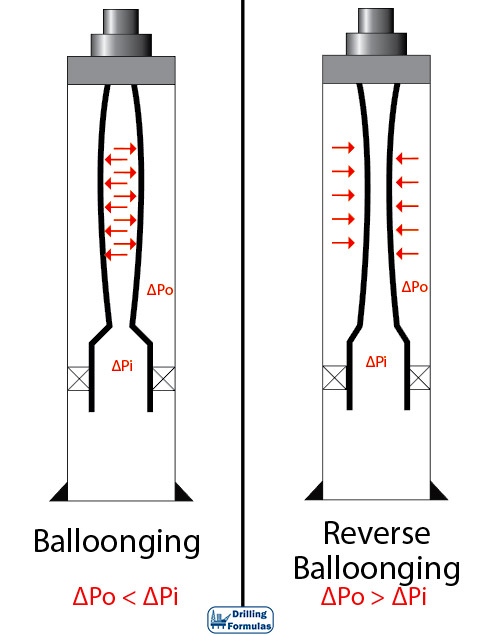Figure 3 -Ballooning and Reverse Ballooning with Pressure Different Diagram

Example

Packer is set at 10,000 ft.

Tubing and packer are free to move.

The well is vertical.

4.5” Tubing

ID of tubing = 3.862”

Packer seal bore outside diameter = 5.0”

Weight per length = 17.7 lb/ft.

E (Young’s modulus) = 30 × 106

µ (Poisson’s ratio) = 0.3

Initial Condition

Fluid in annulus = 10.0 ppg

Fluid in tubing = 10.0 ppg

Tubing pressure = 0 psi

Annulus pressure = 0 psi

Final Condition

Fluid in annulus = 10.0 ppg

Fluid in tubing = 8.0 ppg

Tubing pressure = 1,500 psi

Annulus pressure = 0 psi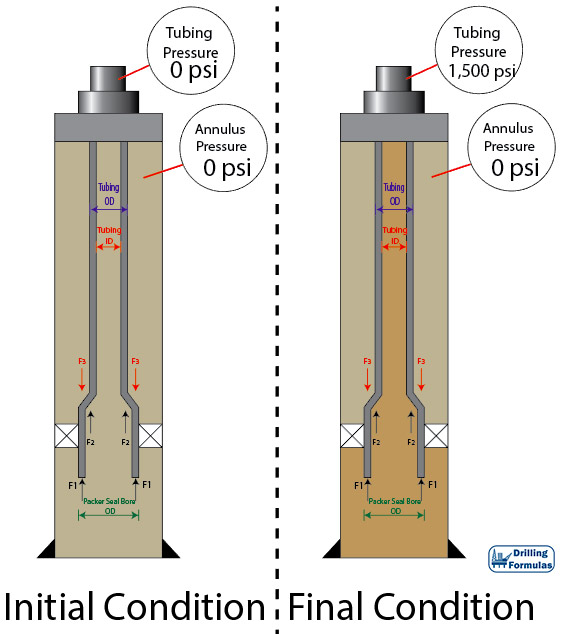Figure 4 – Initial and Final Condition

Solution

For the analysis, Lubinski’s sign conventions are used. Please see at the reference section to get a full paper.

Compression force = ⇑(+)

Tensile force = ⇓(-)

Shorten in Length = (-)

Elongate in length = (+)

Calculate Cros Sectional Areas

Ai = Tubing ID area (in2)

Ai= (π÷4) × 3.8622 = 11.497 in2

Ao = Tubing OD area (in2)

Ao= (π÷4) × 4.52 = 15.904 in2

Determine Average Pressure at Initial Condition

Po @ surface = 0 psi

Pi @ surface = 0 psi

Po @ packer = Po @ surface + Hydrostatic P in annulus = 0 + (0.052×10×10,000) = 5,200 psi

Pi @ packer = Pi @ surface + Hydrostatic P in tubing = 0 + (0.052×10×10,000) = 5,200 psi

Po average @ initial condition = (0 + 5,200) ÷ 2 = 2,600 psi

Pi average@ initial condition = (0 + 5,200) ÷ 2 = 2,600 psi

Determine Average Pressure at Final Condition

Po @ surface = 0 psi

Pi @ surface = 1500 psi

Po @ packer = Po @ surface + Hydrostatic P in annulus = 0 + (0.052×10×10,000) = 5,200 psi

Pi @ packer = Pi @ surface + Hydrostatic P in tubing =1,500 + (0.052×8×10,000) = 5,660 psi

Po average @ final condition = (0 + 5,200) ÷ 2 = 2,600 psi

Pi average @ final condition = (1,500 + 5,660) ÷ 2 = 3,580 psi

Determine Pressure Change (ΔP)

ΔPo = Change in average annulus pressure

ΔPo = 2,600 – 2,600 = 0 psi

ΔPi = Change in average tubing pressure

ΔPi = 3,580 – 2,600 = 980 psi

Length Change due to Ballooning

Length = 10,000 ft. = 120,000 inch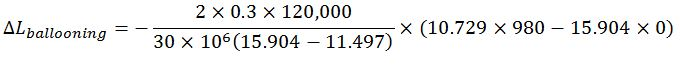ΔLballooning = – 6.14 inches (shorten)

Length change due to ballooning effect is 6.14 inches shorter.

References

Jonathan Bellarby, 2009. Well Completion Design, Volume 56 (Developments in Petroleum Science). 1 Edition. Elsevier Science.

Wan Renpu, 2011. Advanced Well Completion Engineering, Third Edition. 3 Edition. Gulf Professional Publishing.

Ted G. Byrom, 2014. Casing and Liners for Drilling and Completion, Second Edition: Design and Application (Gulf Drilling Guides). 2 Edition. Gulf Professional Publishing.

Lubinski, A., & Althouse, W. S. (1962, June 1). Helical Buckling of Tubing Sealed in Packers. Society of Petroleum Engineers. doi:10.2118/178-PA

Share the joy
Tagged , , , , , . Bookmark the permalink.